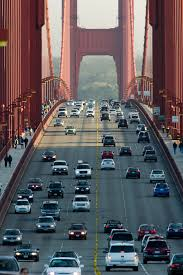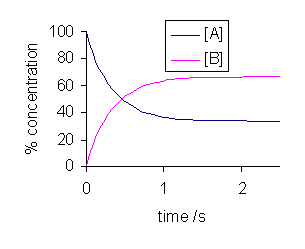## Equilibrium

Chemical equilibrium is the state in which the forward reaction rate and the reverse reaction rate are equal.

### Learning Objectives

Recall the relationship between the forward and reverse reaction rates when a reaction is at equilibrium

### Key Takeaways

#### Key Points

• In a chemical equilibrium, the forward and reverse reactions occur at equal rates, and the concentrations of products and reactants remain constant.
• A catalyst speeds up the rate of a chemical reaction, but has no effect upon the equilibrium position for that reaction.

#### Key Terms

• chemical equilibrium: In a chemical reaction, the state in which both reactants and products are present at concentrations that have no further tendency to change with time.
• the equilibrium position: The point in a chemical reaction at which the concentrations of reactants and products are no longer changing.

In theory, all chemical reactions are in fact double reactions: for every forward reaction, there is a subsequent reverse reaction. The idea can be illustrated as follows:

$\text{reactants}\rightleftharpoons \text{products}$

For plenty of reactions, however, the forward reaction is so favored, and the reverse reaction is so negligible, that reactions are written simply in terms of the solid forward arrow, $\text{A}\rightarrow \text{B}$. However, we will now consider forward/reverse reaction pairs that exist in chemical equilibrium with one another.

### The Concept of Chemical Equilibrium

In a chemical reaction, chemical equilibrium is the state in which the forward reaction rate and the reverse reaction rate are equal. The result of this equilibrium is that the concentrations of the reactants and the products do not change. However, just because concentrations aren’t changing does not mean that all chemical reaction has ceased. Just the opposite is true; chemical equilibrium is a dynamic state in which reactants are being converted into products at all times, but at the exact rate that products are being converted back into reactants. The result of such a situation is analogous to a bridge between two cities, where the rate of cars going over the bridge in each direction is exactly equal. The result is that the net number of cars on either side of the bridge does not change.Cars entering and leaving a city over a bridge: Chemical equilibrium is akin to two cities connected a bridge. If the rate at which cars leave the city is exactly equal to the rate at which cars enter the city, the result is that the net number of cars on both sides of the bridge does not change.

### Reaching Equilibrium and the Equilibrium Position

When a system consists of competing forward and reverse reaction rates, the reaction will proceed until chemical equilibrium is reached. Consider the following general reaction between A and B:

$\text{A}\rightleftharpoons \text{B}$

Let’s say that A and B are introduced into a reaction vessel, and a reaction begins to proceed. The concentrations of A and B will change over time, until they reach equilibrium.Concentration vs. time for a reversible reaction: Note that equilibrium is reached when both the curves plateau, and the concentrations of both reactants and products do not change afterward.

Note that over time, the curves level out, or plateau, and the concentrations of A and B are no longer changing. This is the point at which the system has reached chemical equilibrium. While there are various factors that can increase or decrease the amount of time it takes for a given system to reach equilibrium, the equilibrium position itself is unaffected by these factors. For instance, if a catalyst is added to the system, the reaction will proceed more quickly, and equilibrium will be reached faster, but the concentrations of both A and B will be the same at equilibrium for both the catalyzed and the uncatalyzed reaction.

### Conclusion

The equilibrium state is one in which there is no net change in the concentrations of reactants and products. Despite the fact that there is no apparent change at equilibrium, this does not mean that all chemical reaction has ceased. Nothing could be further from the truth; at equilibrium, the forward and reverse reactions continue, but at identical rates, thereby leaving the net concentrations of reactants and products undisturbed.

Equilibrium lesson: This lesson introduces equilibrium as a condition where the forward and reverse reaction rates are equal. We also explain how the concentrations remain constant at equilibrium.

## The Equilibrium Constant

The thermodynamic equilibrium constant is defined as: $\text{K}_{\text{eq}}=\frac{[\text{C}]^{\text{m}}[\text{D}]^{\text{n}}}{[\text{A}]^{\text{a}}[\text{B}]^{\text{b}}}$.

### Learning Objectives

Duplicate the form of the equation for the thermodynamic equilibrium constant

### Key Takeaways

#### Key Points

• In the equilibrium constant expression, the concentrations of the products go in the numerator and the concentrations of the reactants go the denominator.
• The equilibrium constant is derived from the rate laws for the forward and reverse reactions.
• Only species that exist in the gas or aqueous phases are included in the Keq expression. Reactants and products that exist as solids and liquids are omitted.
• The value of Keq can be used to make qualitative judgments about the thermodynamics of the forward and reverse reactions.

#### Key Terms

• chemical equilibrium: The state of a reversible reaction in which the rates of the forward and reverse reactions are the same.
• activity: Referring to the ideal concentration of a species.

The relationship between forward and reverse reactions in dynamic equilibrium can be expressed mathematically in what is known an equilibrium expression, or Keq expression. Most often, this expression is written in terms of the concentrations of the various reactants and products, and is given by:

$\text{K}_{\text{eq}}=\frac{[\text{C}]^{\text{m}}[\text{D}]^{\text{n}}}{[\text{A}]^{\text{a}}[\text{B}]^{\text{b}}}$

Species in brackets represent the concentrations of products, which are always in the numerator, and reactants, which are always in the denominator. Each of the concentrations is raised to a power equal to the stoichiometric coefficient for each species.

### Derivation of the Equilibrium Expression from the Law of Mass Action

Consider the following general, reversible reaction:

$\text{aA}+\text{bB}\rightleftharpoons \text{mC}+\text{nD}$

Assuming this reaction is an elementary step, we can write the rate laws for both the forward and reverse reactions:

$\text{rate}_{\text{forward}}=\text{k}_1[\text{A}]^\text{a}[\text{B}]^\text{b}$

$\text{rate}_{\text{reverse}}=\text{k}_2[\text{C}]^\text{m}[\text{D}]^\text{n}$

However, we know that the forward and reverse reaction rates are equal in equilibrium:

$\text{k}_1[\text{A}]^\text{a}[\text{B}]^\text{b}=\text{k}_2[\text{C}]^\text{m}[\text{D}]^\text{n}$

Rearranging this equation and separating the rate constants from the concentration terms, we get:

$\frac{\text{k}_1}{\text{k}_2}=\frac{[\text{C}]^\text{m}[\text{D}]^\text{n}}{[\text{A}]^\text{a}[\text{B}]^\text{b}}$

Notice that the left side of the equation is the quotient of two constants, which is simply another constant. We simplify and write this constant as Keq:

$\frac{\text{k}_1}{\text{k}_2}=\text{K}_{\text{C}}=\frac{[\text{C}]^\text{m}[\text{D}]^\text{n}}{[\text{A}]^\text{a}[\text{B}]^\text{b}}$

Keep in mind that the only species that should be included in the Keq expression are reactants and products that exist as gases or are in aqueous solution. Reactants and products in the solid and liquid phases, even if they are involved in the reaction, are not included in the Keq expression, as these species have activities of 1.

“Activity” is a term in physical chemistry used to describe a substance ‘s ideal concentration. The activity for solids and liquids is 1, so they essentially have a constant concentration of 1, and thereby have no effect on the Keq expression. As such, they are omitted.

### Example

Write the Keq expression for the following reaction:

$\text{H}_2\text{O}(\text{g})+\text{C}(\text{s})\rightleftharpoons \text{H}_2(\text{g})+\text{CO}(\text{g})$

The expression would be written as:

$\text{K}_{\text{eq}}=\frac{[\text{H}_2][\text{CO}]}{[\text{C}][\text{H}_2\text{O}]}=\frac{[\text{H}_2][\text{CO}]}{1\times[\text{H}_2\text{O}]}=\frac{[\text{H}_2][\text{CO}]}{[\text{H}_2\text{O}]}$

Note that because it is a solid, the activity of C(s) is 1, and it is omitted from the final K expression.

### Predicting the Direction of a Reaction From the Value of Keq

When looking at the Keq expression, we should notice that it is essentially a ratio relating the concentrations of products to the concentrations of reactants at equilibrium. If we know the value of Keq, we can draw some conclusions about the thermodynamics of the forward and reverse reactions. These conclusions are summarized as follows:

• A Keq value of << 1 is indicative that the reverse reaction is highly favored over the forward reaction, and the concentrations of reactants are much higher than those of the products at equilibrium.
• A Keq value $\approx$ 1 is indicative that the forward and reverse reactions are about equally favorable, for the ratio of concentrations of reactants and products is close to unity.
• A Keq >>1 is indicative that the forward reaction is highly favored over the reverse reaction, and at equilibrium, the concentrations of the products are much greater than those of the reactants.

The equilibrium constant, Keq: This lesson discusses Keq, including the derivation from the law of mass action, and writing/interpreting equilibrium expressions.